# Grover 算法

## 1.简介

### 创建 Oracle

Grover 算法解决了向解决方案状态添加负相位的 oracle。例如，对于任何状态 |x\rangle 的计算基础:

U_\omega|x\rangle = \bigg\{ \begin{aligned} \phantom{-}|x\rangle \quad \text{if} \; x \neq \omega \\ -|x\rangle \quad \text{if} \; x = \omega \\ \end{aligned}

\omega = \text{101} ，我们的 oracle 将得到矩阵:

U_\omega = \begin{bmatrix} 1 & 0 & 0 & 0 & 0 & 0 & 0 & 0 \\ 0 & 1 & 0 & 0 & 0 & 0 & 0 & 0 \\ 0 & 0 & 1 & 0 & 0 & 0 & 0 & 0 \\ 0 & 0 & 0 & 1 & 0 & 0 & 0 & 0 \\ 0 & 0 & 0 & 0 & 1 & 0 & 0 & 0 \\ 0 & 0 & 0 & 0 & 0 & -1 & 0 & 0 \\ 0 & 0 & 0 & 0 & 0 & 0 & 1 & 0 \\ 0 & 0 & 0 & 0 & 0 & 0 & 0 & 1 \\ \end{bmatrix} \begin{aligned} \\ \\ \\ \\ \\ \\ \leftarrow \omega = \text{101}\\ \\ \\ \\ \end{aligned}

Grover 的算法之所以如此强大，是因为它可以很容易地将问题转换为这种形式的预言。有许多计算问题很难找到解，但验证解相对容易。例如，我们可以通过检查满足所有规则来轻松验证数独的解决方案。对于这些问题，我们可以创建一个函数 f ，它接受一个建议的解决方案 x ，并返回 f(x) = 0 ，如果 x 不是一个解决方案(x \neq \omega)，则返回 f(x) = 1 。我们的 oracle 可以被描述为:

U_\omega|x\rangle = (-1)^{f(x)}|x\rangle

oracle 的矩阵是一个对角线矩阵，形式如下:

U_\omega = \begin{bmatrix} (-1)^{f(0)} & 0 & \cdots & 0 \\ 0 & (-1)^{f(1)} & \cdots & 0 \\ \vdots & 0 & \ddots & \vdots \\ 0 & 0 & \cdots & (-1)^{f(2^n-1)} \\ \end{bmatrix}### 振幅放大

t 步骤后，我们将处于 |\psi_t\rangle 状态，其中 |\psi_t\rangle = (U_s U_f)^t | s \rangle

## 2. 示例:2 个量子比特

1. 根据上面的介绍，在 N=4 的情况下，我们有
\theta = \arcsin \frac{1}{2} = \frac{\pi}{6}.
1. 经过 t 步骤，我们有

(U_s U_\omega)^t | s \rangle = \sin \theta_t | \omega \rangle + \cos \theta_t | s' \rangle ,

其中

\theta_t = (2t+1)\theta.
2. 为了得到 | \omega \rangle ，我们需要 \theta_t =\frac{\pi}{2} ，将 \theta=\frac{\pi}{6} 插入到 t=1 的结果中。这意味着在 t=1 旋转后找到了搜索的元素。

Oracle 为 \lvert \omega \rangle = \lvert 11 \rangle

U_\omega | s \rangle = U_\omega \frac{1}{2}\left( |00\rangle + |01\rangle + |10\rangle + |11\rangle \right) = \frac{1}{2}\left( |00\rangle + |01\rangle + |10\rangle - |11\rangle \right).

U_\omega = \begin{bmatrix} 1 & 0 & 0 & 0 \\ 0 & 1 & 0 & 0 \\ 0 & 0 & 1 & 0 \\ 0 & 0 & 0 & -1 \\ \end{bmatrix}H^{\otimes n}|s\rangle = |0\rangle

U_0 \frac{1}{2}\left( \lvert 00 \rangle + \lvert 01 \rangle + \lvert 10 \rangle + \lvert 11 \rangle \right) = \frac{1}{2}\left( \lvert 00 \rangle - \lvert 01 \rangle - \lvert 10 \rangle - \lvert 11 \rangle \right)H^{\otimes n}U_0 H^{\otimes n} = U_s

U_s 的完整电路如下所示:### 2.1 Qiskit 实现

#initialization
import matplotlib.pyplot as plt
import numpy as np

# importing Qiskit
from qiskit import IBMQ, Aer, assemble, transpile
from qiskit import QuantumCircuit, ClassicalRegister, QuantumRegister
from qiskit.providers.ibmq import least_busy

# import basic plot tools
from qiskit.visualization import plot_histogram


n = 2
grover_circuit = QuantumCircuit(n)


def initialize_s(qc, qubits):
"""Apply a H-gate to 'qubits' in qc"""
for q in qubits:
qc.h(q)
return qc

grover_circuit = initialize_s(grover_circuit, [0,1])
grover_circuit.draw()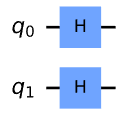|w\rangle = |11\rangle 应用 Oracle。此 oracle 特定于 2 个量子比特:

grover_circuit.cz(0,1) # Oracle
grover_circuit.draw()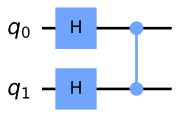# Diffusion operator (U_s)
grover_circuit.h([0,1])
grover_circuit.z([0,1])
grover_circuit.cz(0,1)
grover_circuit.h([0,1])
grover_circuit.draw()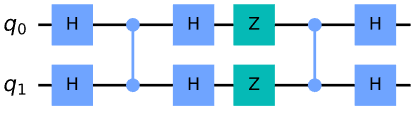### 2.1.1 使用模拟器进行实验

sim = Aer.get_backend('aer_simulator')
# we need to make a copy of the circuit with the 'save_statevector'
# instruction to run on the Aer simulator
grover_circuit_sim = grover_circuit.copy()
grover_circuit_sim.save_statevector()
qobj = assemble(grover_circuit_sim)
result = sim.run(qobj).result()
statevec = result.get_statevector()
from qiskit_textbook.tools import vector2latex
vector2latex(statevec, pretext="|\\psi\\rangle =")

|\psi\rangle =\begin{bmatrix} 0 \\ 0 \\ 0 \\ 1\end{bmatrix}

|11\rangle :

grover_circuit.measure_all()

aer_sim = Aer.get_backend('aer_simulator')
qobj = assemble(grover_circuit)
result = aer_sim.run(qobj).result()
counts = result.get_counts()
plot_histogram(counts)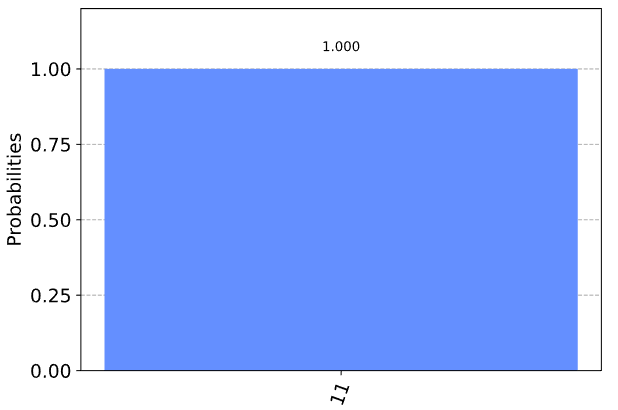### 2.1.2 用真实设备进行实验

# Load IBM Q account and get the least busy backend device
provider = IBMQ.get_provider("ibm-q")
device = least_busy(provider.backends(filters=lambda x: int(x.configuration().n_qubits) >= 3 and
not x.configuration().simulator and x.status().operational==True))
print("Running on current least busy device: ", device)


# Run our circuit on the least busy backend. Monitor the execution of the job in the queue
from qiskit.tools.monitor import job_monitor
transpiled_grover_circuit = transpile(grover_circuit, device, optimization_level=3)
job = device.run(transpiled_grover_circuit)
job_monitor(job, interval=2)


# Get the results from the computation
results = job.result()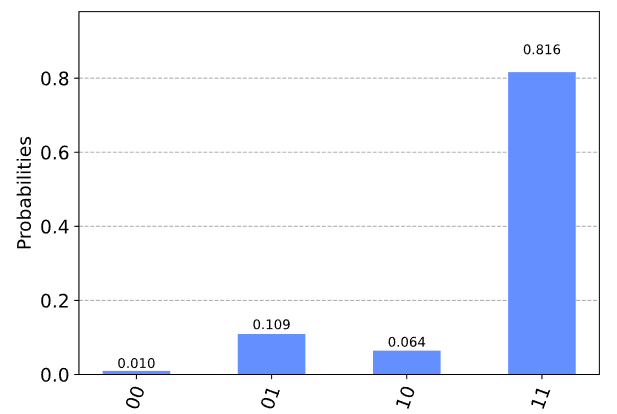## 3.示例:3 个量子比特

1.将哈达玛门应用到初始化为 \lvert000\rangle3 个量子比特，以创建一个统一的叠加:

\lvert \psi_1 \rangle = \frac{1}{\sqrt{8}} \left( \lvert000\rangle + \lvert001\rangle + \lvert010\rangle + \lvert011\rangle + \lvert100\rangle + \lvert101\rangle + \lvert110\rangle + \lvert111\rangle \right)

2.使用相位 oracle 标记 \lvert101\rangle\lvert110\rangle:

\lvert \psi_2 \rangle = \frac{1}{\sqrt{8}} \left( \lvert000\rangle + \lvert001\rangle + \lvert010\rangle + \lvert011\rangle + \lvert100\rangle - \lvert101\rangle - \lvert110\rangle + \lvert111\rangle \right)

3.在平均振幅周围进行反射:

1. 将哈达玛门应用于量子比特
\lvert \psi_{3a} \rangle = \frac{1}{2} \left( \lvert000\rangle +\lvert011\rangle +\lvert100\rangle -\lvert111\rangle \right)
1. 对量子比特应用 X 门
\lvert \psi_{3b} \rangle = \frac{1}{2} \left( -\lvert000\rangle +\lvert011\rangle +\lvert100\rangle +\lvert111\rangle \right)
1. 在 1,2(控制)和 3(目标)量子比特之间应用一个双控制 Z 门
\lvert \psi_{3c} \rangle = \frac{1}{2} \left( -\lvert000\rangle +\lvert011\rangle +\lvert100\rangle -\lvert111\rangle \right)
1. 对量子比特应用 X 门
\lvert \psi_{3d} \rangle = \frac{1}{2} \left( -\lvert000\rangle +\lvert011\rangle +\lvert100\rangle -\lvert111\rangle \right)
1. 对量子比特应用哈达玛门
\lvert \psi_{3e} \rangle = \frac{1}{\sqrt{2}} \left( -\lvert101\rangle -\lvert110\rangle \right)

4.测量 3 个量子比特以检索状态 \lvert101\rangle\lvert110\rangle

### 3.1 Qiskit 实现

qc = QuantumCircuit(3)
qc.cz(0, 2)
qc.cz(1, 2)
oracle_ex3 = qc.to_gate()
oracle_ex3.name = "U$_\omega$"


U_s = H^{\otimes n} U_0 H^{\otimes n}

MCZ = \begin{bmatrix} 1 & 0 & 0 & \cdots & 0 \\ 0 & 1 & 0 & \cdots & 0 \\ \vdots & \vdots & \vdots & \ddots & \vdots \\ 0 & 0 & 0 & \cdots & -1 \\ \end{bmatrix} \begin{aligned} \\ \\ \\ \leftarrow \text{将负相添加到} \; |11\dots 1\rangle\\ \end{aligned}

\begin{aligned} |00\dots 0\rangle & \rightarrow |11\dots 1\rangle\\ |11\dots 1\rangle & \rightarrow |00\dots 0\rangle \end{aligned}

U_0 = - X^{\otimes n} (MCZ) X^{\otimes n}

U_s = - H^{\otimes n} U_0 H^{\otimes n} = H^{\otimes n} X^{\otimes n} (MCZ) X^{\otimes n} H^{\otimes n}

def diffuser(nqubits):
qc = QuantumCircuit(nqubits)
# Apply transformation |s> -> |00..0> (H-gates)
for qubit in range(nqubits):
qc.h(qubit)
# Apply transformation |00..0> -> |11..1> (X-gates)
for qubit in range(nqubits):
qc.x(qubit)
# Do multi-controlled-Z gate
qc.h(nqubits-1)
qc.mct(list(range(nqubits-1)), nqubits-1)  # multi-controlled-toffoli
qc.h(nqubits-1)
# Apply transformation |11..1> -> |00..0>
for qubit in range(nqubits):
qc.x(qubit)
# Apply transformation |00..0> -> |s>
for qubit in range(nqubits):
qc.h(qubit)
# We will return the diffuser as a gate
U_s = qc.to_gate()
U_s.name = "U$_s$"
return U_s


n = 3
grover_circuit = QuantumCircuit(n)
grover_circuit = initialize_s(grover_circuit, [0,1,2])
grover_circuit.append(oracle_ex3, [0,1,2])
grover_circuit.append(diffuser(n), [0,1,2])
grover_circuit.measure_all()
grover_circuit.draw()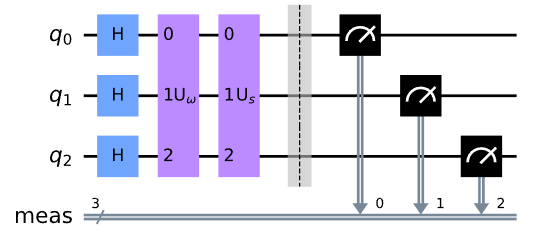### 3.1.1 使用模拟器进行实验

sim = Aer.get_backend('aer_simulator')
# we need to make a copy of the circuit with the 'save_statevector'
# instruction to run on the Aer simulator
grover_circuit_sim = grover_circuit.copy()
grover_circuit_sim.save_statevector()
qobj = assemble(grover_circuit_sim)
result = sim.run(qobj).result()
statevec = result.get_statevector()
from qiskit_textbook.tools import vector2latex
vector2latex(statevec, pretext="|\\psi\\rangle =")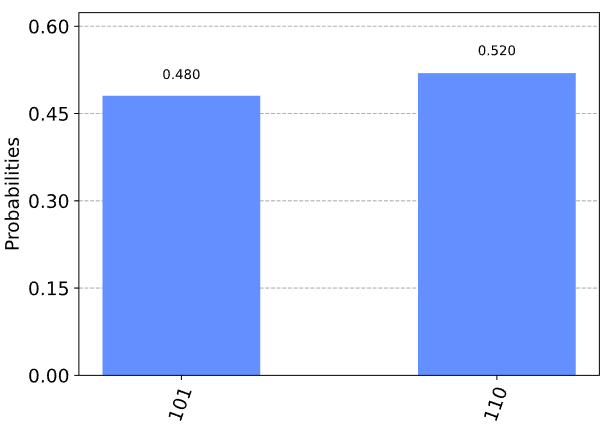### 3.1.2 用真实设备进行实验

backend = least_busy(provider.backends(filters=lambda x: int(x.configuration().n_qubits) >= 3 and
not x.configuration().simulator and x.status().operational==True))
print("least busy backend: ", backend)


# 在最不繁忙的后端运行我们的电路。监控队列中作业的执行
from  qiskit.tools.monitor  import  job_monitor
transpiled_grover_circuit  =  transpile ( grover_circuit ,  device ,  optimization_level = 3 )
job  =  device 。运行( transpiled_grover_circuit )
job_monitor ( job ,  interval = 2 )


# Get the results from the computation
results = job.result()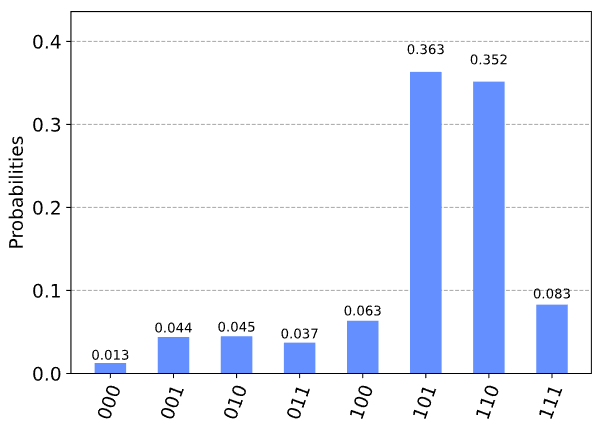## 4. 问题

from qiskit_textbook.problems import grover_problem_oracle
## Example Usage
n = 4
oracle = grover_problem_oracle(n, variant=1)  # 0th variant of oracle, with n qubits
qc = QuantumCircuit(n)
qc.append(oracle, [0,1,2,3])
qc.draw()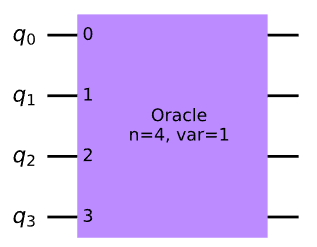1. Grover_problem_oracle (4, variant=2)使用 4 个量子比特，有 1 个解决方案。
a.我们需要多少次迭代才能有 > 90% 的机会测量此解决方案？
b.使用 Grover 算法找到此解决方案状态。
C.如果我们对上面问题 1a 中计算的次数进行更多迭代，会发生什么情况？为什么？

2. 有 2 个解决方案和 4 个量子比特，我们需要多少次迭代才能有>90%的机会测量一个解决方案?使用 oracle grover_problem_oracle(4, variant=1)(它有两个解决方案)测试您的答案。

3. 创建一个函数 grover_solver(oracle, iterations)，将其作为输入:

• Grover oracle 作为门 ( oracle)
• 迭代次数为整数(iterations)并返回一个量子电路，在oracle门上执行 Grover 算法，迭代次数为iterations

## 5. 使用 Grover 算法求解数独

• 任何列都不能两次包含相同的值
• 任何行都不能两次包含相同的值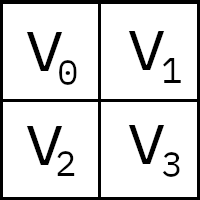### 5.1 把问题变成电路

v0 ≠ v1 # 沿着上面一行检查
v2 ≠ v3 # 沿着下面一行检查
v0 ≠ v2 # 沿着左面一行检查
v1 ≠ v3 # 沿着右面一行检查

clause_list = [[0,1],
[0,2],
[1,3],
[2,3]]


def XOR(qc, a, b, output):
qc.cx(a, output)
qc.cx(b, output)


# We will use separate registers to name the bits
in_qubits = QuantumRegister(2, name='input')
out_qubit = QuantumRegister(1, name='output')
qc = QuantumCircuit(in_qubits, out_qubit)
XOR(qc, in_qubits, in_qubits, out_qubit)
qc.draw()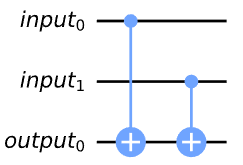# Create separate registers to name bits
var_qubits = QuantumRegister(4, name='v')  # variable bits
clause_qubits = QuantumRegister(4, name='c')  # bits to store clause-checks

# Create quantum circuit
qc = QuantumCircuit(var_qubits, clause_qubits)

# Use XOR gate to check each clause
i = 0
for clause in clause_list:
XOR(qc, clause, clause, clause_qubits[i])
i += 1

qc.draw()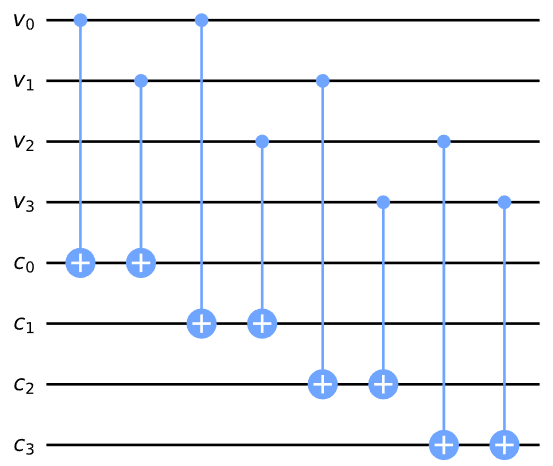# Create separate registers to name bits
var_qubits = QuantumRegister(4, name='v')
clause_qubits = QuantumRegister(4, name='c')
output_qubit = QuantumRegister(1, name='out')
qc = QuantumCircuit(var_qubits, clause_qubits, output_qubit)

# Compute clauses
i = 0
for clause in clause_list:
XOR(qc, clause, clause, clause_qubits[i])
i += 1

# Flip 'output' bit if all clauses are satisfied
qc.mct(clause_qubits, output_qubit)

qc.draw()


### 5.2 取消计算并完成 Oracle

• 一个存储我们的数独变量的寄存器（我们会说 x = v_3, v_2, v_1, v_0 )
• 一个存储我们的子句的寄存器（从状态开始 |0000\rangle 我们将缩写为 |0\rangle )
• 还有一个量子比特（ |\text{out}_0\rangle ) 我们用它来存储校验电路的输出。

U_\omega|x\rangle|0\rangle|\text{out}_0\rangle = |x\rangle|0\rangle|\text{out}_0\oplus f(x)\rangle

\begin{aligned} U_\omega|x\rangle|0\rangle|{-}\rangle &= U_\omega|x\rangle|0\rangle\otimes\tfrac{1}{\sqrt{2}}(|0\rangle - |1\rangle)\\ &= |x\rangle|0\rangle\otimes\tfrac{1}{\sqrt{2}}(|0\oplus f(x)\rangle - |1\oplus f(x)\rangle) \end{aligned}

\begin{aligned} &= |x\rangle|0\rangle\otimes \tfrac{1}{\sqrt{2}}(|0\rangle - |1\rangle)\\ &= |x\rangle|0\rangle|-\rangle\\ \end{aligned}

(即没有变化)。但如果 f(x) = 1 (即 x = \omega )，我们将一个负相位引入 |{-}\rangle 量子比特:

\begin{aligned} &= \phantom{-}|x\rangle|0\rangle\otimes\tfrac{1}{\sqrt{2}}(|1\rangle - |0\rangle)\\ &= \phantom{-}|x\rangle|0\rangle\otimes -\tfrac{1}{\sqrt{2}}(|0\rangle - |1\rangle)\\ &= -|x\rangle|0\rangle|-\rangle\\ \end{aligned}

U_\omega|x\rangle|0\rangle|{-}\rangle = \Bigg\{ \begin{aligned} \phantom{-}|x\rangle|0\rangle|-\rangle \quad \text{for} \; x \neq \omega \\ -|x\rangle|0\rangle|-\rangle \quad \text{for} \; x = \omega \\ \end{aligned}

var_qubits = QuantumRegister(4, name='v')
clause_qubits = QuantumRegister(4, name='c')
output_qubit = QuantumRegister(1, name='out')
cbits = ClassicalRegister(4, name='cbits')
qc = QuantumCircuit(var_qubits, clause_qubits, output_qubit, cbits)

def sudoku_oracle(qc, clause_list, clause_qubits):
# Compute clauses
i = 0
for clause in clause_list:
XOR(qc, clause, clause, clause_qubits[i])
i += 1

# Flip 'output' bit if all clauses are satisfied
qc.mct(clause_qubits, output_qubit)

# Uncompute clauses to reset clause-checking bits to 0
i = 0
for clause in clause_list:
XOR(qc, clause, clause, clause_qubits[i])
i += 1

sudoku_oracle(qc, clause_list, clause_qubits)
qc.draw()


U_\omega|x\rangle|0\rangle|\text{out}_0\rangle = \Bigg\{ \begin{aligned} |x\rangle|0\rangle|\text{out}_0\rangle \quad \text{for} \; x \neq \omega \\ |x\rangle|0\rangle\otimes X|\text{out}_0\rangle \quad \text{for} \; x = \omega \\ \end{aligned}

U_\omega|x\rangle|0\rangle|{-}\rangle = \Bigg\{ \begin{aligned} \phantom{-}|x\rangle|0\rangle|-\rangle \quad \text{for} \; x \neq \omega \\ -|x\rangle|0\rangle|-\rangle \quad \text{for} \; x = \omega \\ \end{aligned}

### 5.3 完整算法

var_qubits = QuantumRegister(4, name='v')
clause_qubits = QuantumRegister(4, name='c')
output_qubit = QuantumRegister(1, name='out')
cbits = ClassicalRegister(4, name='cbits')
qc = QuantumCircuit(var_qubits, clause_qubits, output_qubit, cbits)

# Initialize 'out0' in state |->
qc.initialize([1, -1]/np.sqrt(2), output_qubit)

# Initialize qubits in state |s>
qc.h(var_qubits)
qc.barrier()  # for visual separation

## First Iteration
# Apply our oracle
sudoku_oracle(qc, clause_list, clause_qubits)
qc.barrier()  # for visual separation
# Apply our diffuser
qc.append(diffuser(4), [0,1,2,3])

## Second Iteration
sudoku_oracle(qc, clause_list, clause_qubits)
qc.barrier()  # for visual separation
# Apply our diffuser
qc.append(diffuser(4), [0,1,2,3])

# Measure the variable qubits
qc.measure(var_qubits, cbits)

qc.draw(fold=-1)


# Simulate and plot results
aer_simulator = Aer.get_backend('aer_simulator')
transpiled_qc = transpile(qc, aer_simulator)
qobj = assemble(transpiled_qc)
result = aer_sim.run(qobj).result()
plot_histogram(result.get_counts())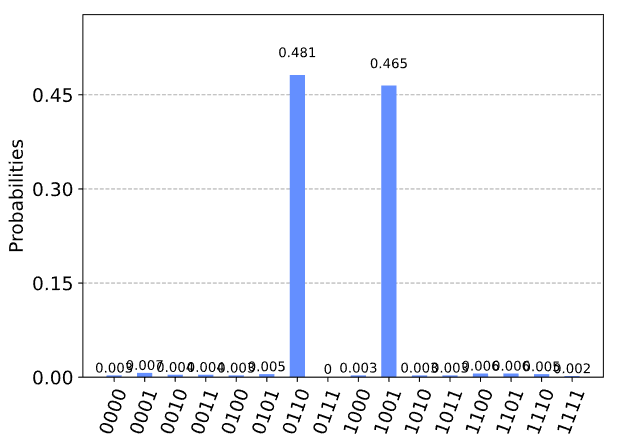v0 = 0
v1 = 1
v2 = 1
v3 = 0

v0 = 1
v1 = 0
v2 = 0
v3 = 1

1. 创建一个可以识别正确解决方案的可逆经典电路

2. 使用相位反冲和未计算将此电路变成一个 oracle

3. 使用Grover的算法来解决这个oracle

## 6. 参考文献

1. L. K. Grover (1996), “A fast quantum mechanical algorithm for database search”, Proceedings of the 28th Annual ACM Symposium on the Theory of Computing (STOC 1996), doi:10.1145/237814.237866, arXiv:quant-ph/9605043

2. C. Figgatt, D. Maslov, K. A. Landsman, N. M. Linke, S. Debnath & C. Monroe (2017), “Complete 3-Qubit Grover search on a programmable quantum computer”, Nature Communications, Vol 8, Art 1918, doi:10.1038/s41467-017-01904-7, arXiv:1703.10535

3. I. Chuang & M. Nielsen, “Quantum Computation and Quantum Information”, Cambridge: Cambridge University Press, 2000.

import qiskit.tools.jupyter
%qiskit_version_table


### 版本信息

Qiskit Software Version
Qiskit 0.27.0
Terra 0.17.4
Aer 0.8.2
Ignis 0.6.0
Aqua 0.9.2
IBM Q Provider 0.14.0
System information
Python 3.7.7 (default, May 6 2020, 04:59:01) [Clang 4.0.1 (tags/RELEASE_401/final)]
OS Darwin
CPUs 8
Memory (Gb) 32.0
Wed Jun 16 13:45:01 2021 BST